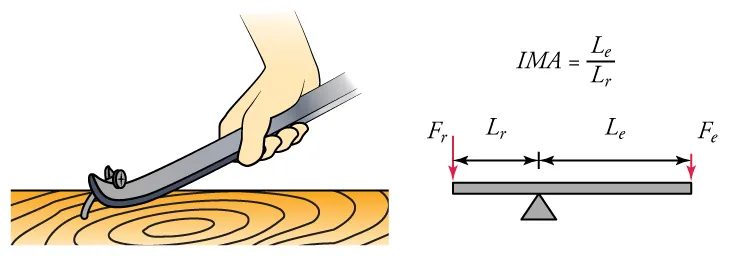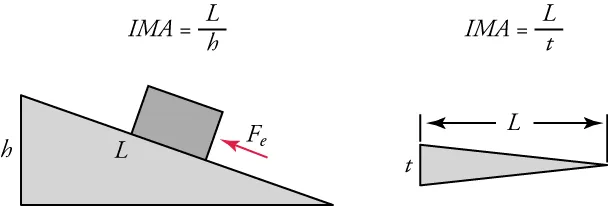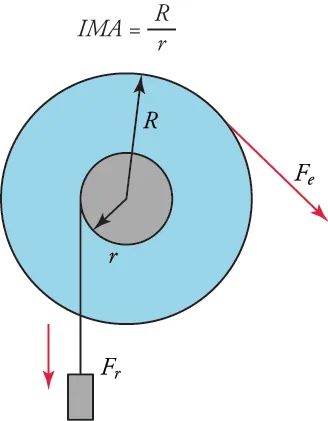Physics

#### 9.1Work, Power, and the Work–Energy Theorem

34 .
Describe two ways in which doing work on an object can increase its mechanical energy.
1. Raising an object to a higher elevation does work as it increases its PE; increasing the speed of an object does work as it increases its KE.
2. Raising an object to a higher elevation does work as it increases its KE; increasing the speed of an object does work as it increases its PE.
3. Raising an object to a higher elevation does work as it increases its PE; decreasing the speed of an object does work as it increases its KE.
4. Raising an object to a higher elevation does work as it increases its KE; decreasing the speed of an object does work as it increases its PE.
35.

True or false—While riding a bicycle up a gentle hill, it is fairly easy to increase your potential energy, but to increase your kinetic energy would make you feel exhausted.

1. True
2. False
36.

Which statement best explains why running on a track with constant speed at 3 m/s is not work, but climbing a mountain at 1 m/s is work?

1. At constant speed, change in the kinetic energy is zero but climbing a mountain produces change in the potential energy.
2. At constant speed, change in the potential energy is zero, but climbing a mountain produces change in the kinetic energy.
3. At constant speed, change in the kinetic energy is finite, but climbing a mountain produces no change in the potential energy.
4. At constant speed, change in the potential energy is finite, but climbing a mountain produces no change in the kinetic energy.
37 .
You start at the top of a hill on a bicycle and coast to the bottom without applying the brakes. By the time you reach the bottom of the hill, work has been done on you and your bicycle, according to the equation: $W = \frac{1}{2}m(v_2^{\,2} - v_1^{\,2})$ If $m$ is the mass of you and your bike, what are ${v_1}\!$ and ${v_2}$?
1. ${v_1}$ is your speed at the top of the hill, and ${v_2}$ is your speed at the bottom.
2. ${v_1}$ is your speed at the bottom of the hill, and ${v_2}$ is your speed at the top.
3. ${v_1}$ is your displacement at the top of the hill, and ${v_2}$ is your displacement at the bottom.
4. ${v_1}$ is your displacement at the bottom of the hill, and ${v_2}$ is your displacement at the top.

#### 9.2Mechanical Energy and Conservation of Energy

38.

True or false—The formula for gravitational potential energy can be used to explain why joules, J, are equivalent to kg × mg2 / s2 . Show your work.

1. True
2. False
39 .
Which statement best explains why accelerating a car from $20\,\text{mph}$ to $40\,\text{mph}$ quadruples its kinetic energy?
1. Because kinetic energy is directly proportional to the square of the velocity.
2. Because kinetic energy is inversely proportional to the square of the velocity.
3. Because kinetic energy is directly proportional to the fourth power of the velocity.
4. Because kinetic energy is inversely proportional to the fourth power of the velocity.
40 .
A coin falling through a vacuum loses no energy to friction, and yet, after it hits the ground, it has lost all its potential and kinetic energy. Which statement best explains why the law of conservation of energy is still valid in this case?
1. When the coin hits the ground, the ground gains potential energy that quickly changes to thermal energy.
2. When the coin hits the ground, the ground gains kinetic energy that quickly changes to thermal energy.
3. When the coin hits the ground, the ground gains thermal energy that quickly changes to kinetic energy.
4. When the coin hits the ground, the ground gains thermal energy that quickly changes to potential energy.
41.

True or false—A marble rolls down a slope from height h1 and up another slope to height h2, where (h2 < h1). The difference mg(h1h2) is equal to the heat lost due to the friction.

1. True
2. False

#### 9.3Simple Machines

42.

Why would you expect the lever shown in the top image to have a greater efficiency than the inclined plane shown in the bottom image?1. The resistance arm is shorter in case of the inclined plane.
2. The effort arm is shorter in case of the inclined plane.
3. The area of contact is greater in case of the inclined plane.
43.

Why is the wheel on a wheelbarrow not a simple machine in the same sense as the simple machine in the image?1. The wheel on the wheelbarrow has no fulcrum.
2. The center of the axle is not the fulcrum for the wheels of a wheelbarrow.
3. The wheelbarrow differs in the way in which load is attached to the axle.
4. The wheelbarrow has less resistance force than a wheel and axle design.
44.

A worker pulls down on one end of the rope of a pulley system with a force of 75 N to raise a hay bale tied to the other end of the rope. If she pulls the rope down 2.0 m and the bale raises 1.0 m, what else would you have to know to calculate the efficiency of the pulley system?

1. the weight of the worker
2. the weight of the hay bale
3. the radius of the pulley
4. the height of the pulley from ground
45.

True or false—A boy pushed a box with a weight of 300 N up a ramp. He said that, because the ramp was 1.0 m high and 3.0 m long, he must have been pushing with force of exactly 100 N.

1. True
2. False
Order a print copy

As an Amazon Associate we earn from qualifying purchases.